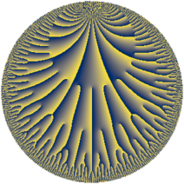# Properties

 Label 25.34.dLevel $25$ Weight $34$ Character orbit 25.d Rep. character $\chi_{25}(6,\cdot)$ Character field $\Q(\zeta_{5})$ Dimension $324$ Sturm bound $85$

# Related objects

## Defining parameters

 Level: $$N$$ $$=$$ $$25 = 5^{2}$$ Weight: $$k$$ $$=$$ $$34$$ Character orbit: $$[\chi]$$ $$=$$ 25.d (of order $$5$$ and degree $$4$$) Character conductor: $$\operatorname{cond}(\chi)$$ $$=$$ $$25$$ Character field: $$\Q(\zeta_{5})$$ Sturm bound: $$85$$

## Dimensions

The following table gives the dimensions of various subspaces of $$M_{34}(25, [\chi])$$.

Total New Old
Modular forms 332 332 0
Cusp forms 324 324 0
Eisenstein series 8 8 0

## Trace form

 $$324q + 65533q^{2} - 87364951q^{3} - 339302416387q^{4} - 636960114110q^{5} + 5659399684093q^{6} + 180644889073248q^{7} - 1507000349560340q^{8} - 139598066558332528q^{9} + O(q^{10})$$ $$324q + 65533q^{2} - 87364951q^{3} - 339302416387q^{4} - 636960114110q^{5} + 5659399684093q^{6} + 180644889073248q^{7} - 1507000349560340q^{8} - 139598066558332528q^{9} - 53376759697000755q^{10} + 392543399750177983q^{11} + 1280853451713570283q^{12} + 4969115442381785109q^{13} + 18409493073831919231q^{14} + 98845717093944245025q^{15} - 1111631449875979388111q^{16} + 541697218613260133813q^{17} - 4645186806981592016976q^{18} + 603918334415854145485q^{19} + 7395898404333976225895q^{20} + 15774370344809451307488q^{21} - 60938480418058436839784q^{22} - 47948670368089662380111q^{23} - 214884441704807705380260q^{24} + 222026125113718008621220q^{25} - 959644399464272082946722q^{26} + 230498155582457733344195q^{27} - 5175332465617983003004389q^{28} + 4482533322744929530310675q^{29} + 6492399624480264998557035q^{30} - 6440819317072818189184167q^{31} - 56901293114542301634677802q^{32} + 66269267902602884153696363q^{33} + 11218783919155715446947821q^{34} + 8067403069902432695465690q^{35} - 484230535836298348191716531q^{36} - 100860018401894767851608067q^{37} - 297508256642047715200493635q^{38} - 16530761145795321381533741q^{39} - 2562355220293846799087107470q^{40} - 565567410715289862616950367q^{41} - 4372282018924939100563252579q^{42} + 3100310984254670532970195044q^{43} - 4814450630372787380056206084q^{44} + 16206153359119737990724625600q^{45} + 14530299338609028002865059033q^{46} - 16199052109998741254491929857q^{47} + 41990666227001543189136273994q^{48} + 332164331941026159327717146448q^{49} + 33796109071678434514582637165q^{50} - 19367926877179854098166762922q^{51} + 87530358607023900215474594568q^{52} + 19171827629834195922431377959q^{53} + 23198025460722196640564934895q^{54} - 243980769279848480374699869815q^{55} + 7445090671537796892534552810q^{56} - 1057226344955597095488539882930q^{57} + 588361759674313791483013220830q^{58} + 74689935521459956100735927895q^{59} - 1769886073905535539385864025190q^{60} - 300079345995407374248814590017q^{61} + 1409441265746697878478345620856q^{62} - 1303504279815381305677668585166q^{63} - 2423164453984407030615106332212q^{64} + 1735302665887068485829374022705q^{65} + 3423409463111573143266624240776q^{66} + 202423586255713471493267530853q^{67} - 13937250281224604264259751294834q^{68} + 5264371378103678515981166207639q^{69} + 13731503278313676874117177195880q^{70} + 9210737490073137056800208212073q^{71} - 21403095253310438833803549460895q^{72} + 16579732345699934918005410941109q^{73} - 64936241000699136664131624429804q^{74} + 4413188525069649637208068633295q^{75} - 104094823992544958247975163849280q^{76} - 53780742940648963685437473223144q^{77} + 99951922938852848302909346380913q^{78} + 77948888580679597722934213075175q^{79} - 251259603793182242054832153168420q^{80} - 398782124631112906155074871667666q^{81} + 703784722592430736227126449236306q^{82} + 106614345829501107264310341232209q^{83} - 667332683303589414657079617068044q^{84} + 233114537042673971093605494229665q^{85} + 759969567850007046672226801727103q^{86} + 606374452061689985810020353660995q^{87} + 1264254698822866575161055232420320q^{88} - 494981772860954156567631250157885q^{89} + 2560303450946569952633917555542655q^{90} + 833380852148569239280599836950568q^{91} + 151629397723296769588686938126568q^{92} + 3304740515444009381235293446628878q^{93} + 1400671517764476918805870186870901q^{94} - 1280128196628161102416301552151455q^{95} + 820755579419642667336362813280838q^{96} + 2952047119943346577626041361334303q^{97} - 2540357364753245529290787480239344q^{98} - 4926798503343641962968338609297476q^{99} + O(q^{100})$$

## Decomposition of $$S_{34}^{\mathrm{new}}(25, [\chi])$$ into newform subspaces

The newforms in this space have not yet been added to the LMFDB.